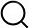# Also in the Article

Microscopy

Procedure

To derive the gap between the surfaces through the intensity, the confocal microscope was turned into reflection mode. Reflection of the laser from the bead surface forms an interference pattern known as Newton rings. The maximal intensity of the reflection will occur when the distance between the surfaces is$d=(m+12)λ2n$(2)where m = 0, 1, 2, 3, … is the ring number, λ = 514 nm is the excitation wavelength, and n = 1.484 is the refractive index of the fluorescent liquid. Thus, correlation between intensity and thickness is obtained by taking and comparing a cross section of the fluorescence intensity image and that of the laser reflection image. For details, see (19).

Q&A